International
Tables for
Crystallography
Volume D
Physical properties of crystals
Edited by A. Authier

International Tables for Crystallography (2006). Vol. D, ch. 1.1, pp. 15-16

## Section 1.1.4.6.2.3. General case

A. Authiera*

aInstitut de Minéralogie et de la Physique des Milieux Condensés, Bâtiment 7, 140 rue de Lourmel, 75015 Paris, France
Correspondence e-mail: aauthier@wanadoo.fr

#### 1.1.4.6.2.3. General case

| top | pdf |

By replacing the matrix coefficients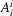by their expression, (1.1.4.2)becomes, for a proper rotation,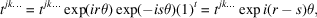where r is the number of indices equal to 1, s is the number of indices equal to 2, t is the number of indices equal to 3 and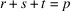is the rank of the tensor. The component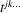is not affected by the symmetry operation if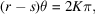where K is an integer, and is equal to zero if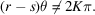The angle of rotation θ can be put into the form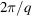, where q is the order of the axis. The condition for the component not to be zero is then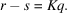The condition is fulfilled differently depending on the rank of the tensor, p, and the order of the axis, q. Indeed, we have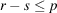and,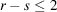: the result of the reduction will be the same for any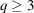;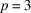,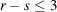: the result of the reduction will be the same for any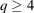;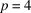,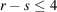: the result of the reduction will be the same for any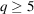.

It follows that:

 (i) for tensors of rank 2, the reduction will be the same for trigonal (threefold axis), tetragonal (fourfold axis) and hexagonal (sixfold axis) groups; (ii) for tensors of rank 3, the reduction will be the same for tetragonal and hexagonal groups; (iii) for tensors of rank 4, the reduction will be different for trigonal, tetragonal and hexagonal groups.

The inconvenience of the diagonalization method is that the vectors and eigenvalues are, in general, complex, so in practice one uses another method. For instance, we may note that equation (1.1.4.1)can be written in the case ofby associating with the tensor amatrix T: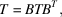where B is the symmetry operation. Through identification of homologous coefficients in matrices T and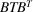, one obtains relations between components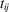that enable the determination of the independent components.